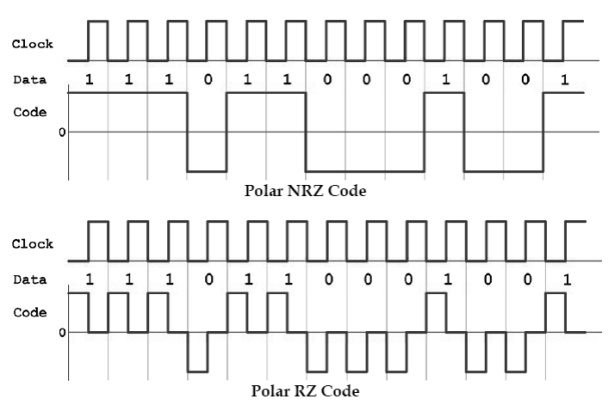Home | | Digital Communication | Power spectral density of unipolar NRZ line code

# Power spectral density of unipolar NRZ line code

Line coding: Line coding refers to the process of representing the bit stream (1s and 0s) in the form of voltage or current variations optimally tuned for the specific propertiesof the physical channel being used.

Power spectral density of unipolar NRZ line code:

## Line coding:

Line coding refers to the process of representing the bit stream (1s and 0s) in the form of voltage or current variations optimally tuned for the specific propertiesof the physical channel being used. The selection of a proper line code can help in so many ways: One possibility is to aid in clock recovery at the receiver. A clock signal is recovered by observing transitions in the received bit sequence, and if enough transitions exist, a good recovery of the clock is guaranteed, and the signal is said to be self-clocking.

Another advantage is to get rid of DC shifts. The DC component in a line code is called the bias or the DC coefficient. Unfortunately, most long-distance communication channels cannot transport a DC component. This is why most line codes try to eliminate the DC component before being transmitted on the channel. Such codes are called DCbalanced, zero-DC, zero-bias, or DC equalized. Some common types of line encodingin common-use nowadays are unipolar, polar, bipolar, Manchester, MLT-3 and Duobinary encoding. These codes are explained here: 1. Unipolar(Unipolar NRZ and Unipolar RZ):

Unipolar is the simplest line coding scheme possible. It has the advantage of being compatible with TTL logic. Unipolar coding uses a positive rectangular pulse p(t) to represent binary 1, and the absence of a pulse (i.e., zero voltage) to represent a binary 0. Two possibilities for the pulsep(t) exist3: Non-Return-to-Zero (NRZ) rectangularpulse and Return-to-Zero (RZ) rectangular pulse. The difference between Unipolar NRZ and Unipolar RZ codes is that the rectangular pulse in NRZ stays at a positive value (e.g., +5V) for the full duration of the logic 1 bit, while the pule in RZ drops from +5V to 0V in the middle of the bit time. A drawback of unipolar (RZ and NRZ) is that its average value is not zero, which means it creates a significant DC-component at the receiver (see the impulse at zero frequency in the corresponding power spectral density (PSD) of this line code.that polar signals have more power than unipolar signals, and hence have better SNR at the receiver. Actually, polar NRZ signals have more power compared to polar RZ signals. The drawback of polar NRZ, however, is that it lacks clock information especially when a long sequence of 0‟s or 1‟s is transmitted.

Non-Return-to-Zero, Inverted (NRZI): NRZI is a variant of Polar NRZ. In NRZI there aretwo possible pulses, p(t) and –p(t). A transition from one pulse to the other happens if the bit being transmitted is logic 1, and no transition happens if the bit being transmitted is a logic 0.This is the code used on compact discs (CD), USB ports, and on fiber-based Fast Ethernet at 100-Mbit/s.

Study Material, Lecturing Notes, Assignment, Reference, Wiki description explanation, brief detail
Digital Communication : Baseband Transmission : Power spectral density of unipolar NRZ line code |